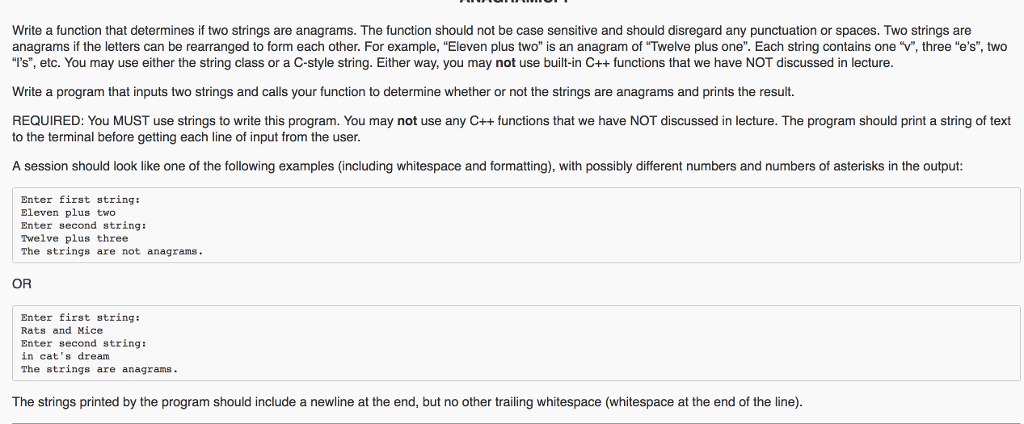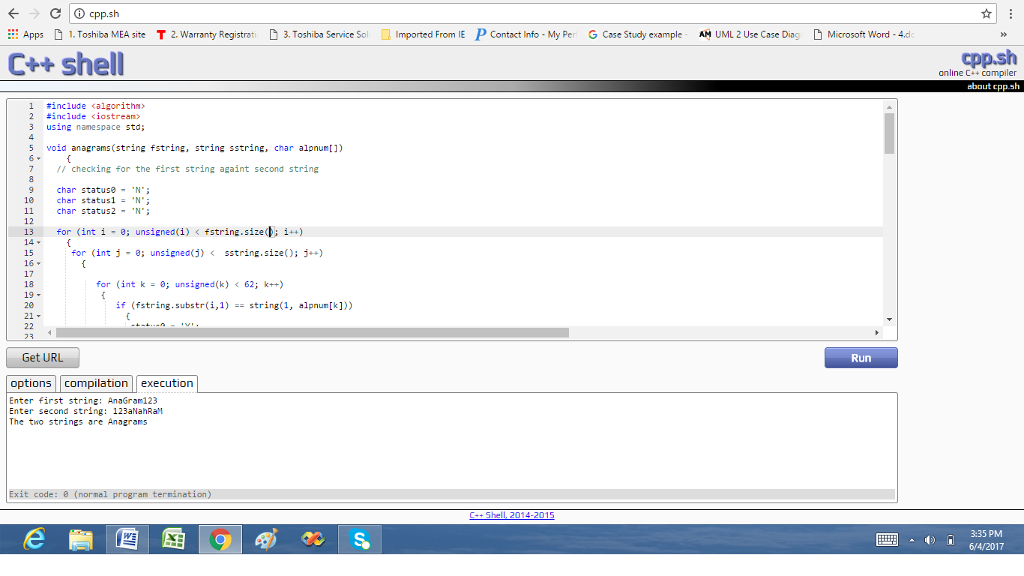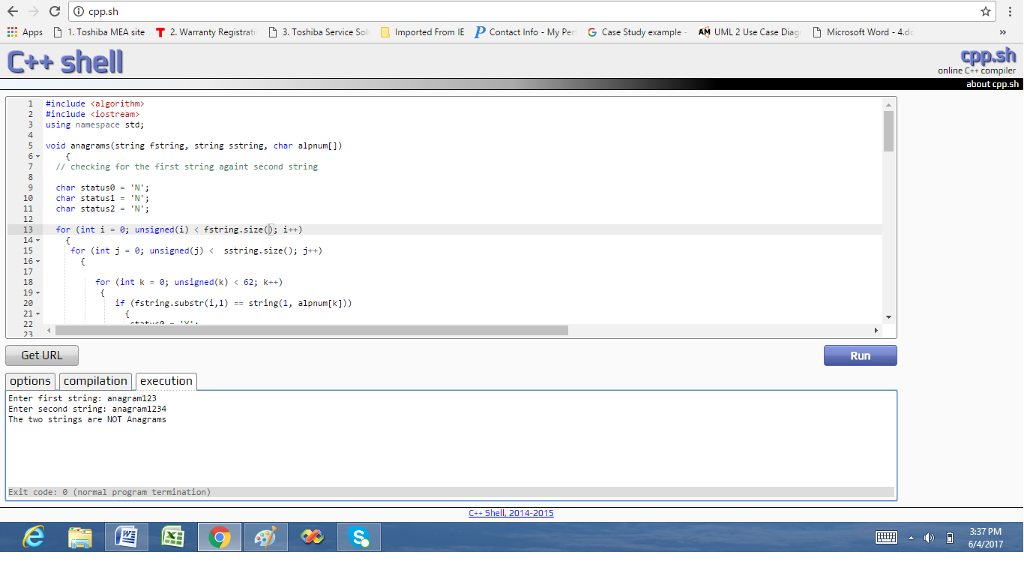# Answered! Write a function that determines if two strings are anagrams. The function should not be case sensitive and should…

In C++Write a function that determines if two strings are anagrams. The function should not be case sensitive and should disregard any punctuation or spaces. Two strings are anagrams if the letters can be rearranged to form each other. For example, “Eleven plus two” is an anagram of “Twelve plus one”. Each string contains one “v”, three “e’s”, two “l’s”, etc. You may use either the string class or a C-style string. Either way, you may not use built-in C++ functions that we have NOT discussed in lecture. Write a program that inputs two strings and calls your function to determine whether or not the strings are anagrams and prints the result. REQUIRED: You MUST use strings to write this program. You may not use any C++ functions that we have NOT discussed in lecture. The program should print a string of text to the terminal before getting each line of input from the user. A session should look like one of the following examples (including whitespace and formatting), with possibly different numbers and numbers of asterisks in the output: Enter first string: Eleven plus two Enter second string: Twelve plus three The strings are not anagrams. OR Enter first string: Rats and Mice Enter second string: in cat’s dream The strings are anagrams. The strings printed by the program should include a newline at the end, but no other trailing whitespace (whitespace at the end of the line).

#include <algorithm>
#include <iostream>
using namespace std;

void anagrams(string fstring, string sstring, char alpnum[])
{
// checking for the first string againt second string

Don't use plagiarized sources. Get Your Custom Essay on
Answered! Write a function that determines if two strings are anagrams. The function should not be case sensitive and should…
GET AN ESSAY WRITTEN FOR YOU FROM AS LOW AS \$13/PAGE

char status0 = ‘N’;
char status1 = ‘N’;
char status2 = ‘N’;

for (int i = 0; unsigned(i) < fstring.size(); i++)
{
for (int j = 0; unsigned(j) < sstring.size(); j++)
{

for (int k = 0; unsigned(k) < 62; k++)
{
if (fstring.substr(i,1) == string(1, alpnum[k]))
{
status0 = ‘Y’;
}
}

if (status0 == ‘Y’)
{
if (fstring.substr(i,1) == sstring.substr(j,1))
{
status1 = ‘Y’;
j = sstring.size();
}else{
status1 = ‘N’;
}
}
if (status1 == ‘Y’)
{
status2 = ‘Y’;
}else{
status2 = ‘N’;
}
}
}

// checking the secodn string against first string

for (int i2 = 0; unsigned(i2) < sstring.size(); i2++)
{
for (int j2 = 0; unsigned(j2) < fstring.size(); j2++)
{

for (int k2 = 0; unsigned(k2) < 62; k2++)
{
if (sstring.substr(i2,1) == string(1, alpnum[k2]))
{
status0 = ‘Y’;
}
}

if (status0 == ‘Y’)
{
if (sstring.substr(i2,1) == fstring.substr(j2,1))
{
status1 = ‘Y’;
j2 = fstring.size();
}else{
status1 = ‘N’;
}
}
if (status1 == ‘Y’)
{
status2 = ‘Y’;
}else{
status2 = ‘N’;
}
}
}

// for both conditions satisfy accept as Anagrams

if (status2 == ‘Y’)
{
cout << “The two strings are Anagrams” << “n”;
}else{
cout << “The two strings are NOT Anagrams” << “n”;
}
}

int main()
{
string fstring;
string sstring;
char alpnum[] = {‘a’,’b’,’c’,’d’,’e’,’f’,’g’,’h’,’i’,’j’,’k’,’l’,’m’,’n’,’o’,’p’,’q’,’r’,’s’,’t’,’u’,’v’,’w’,’x’,’y’,’z’,’A’,’B’,’C’,’D’,’E’,’F’,’G’,’H’,’I’,’J’,’K’,’L’,’M’,’N’,’O’,’P’,’Q’,’R’,’S’,’T’,’U’,’V’,’W’,’X’,’Y’,’Z’,’0′,’1′,’2′,’3′,’4′,’5′,’6′,’7′,’8′,’9′};
// array used due to isalnum(), isalph() and isdigit() not working, therefore for checking alpha numeric charector only

void anagrams(string fstring, string sstring, char alpnum[]);
cout << “Enter first string: “;
cin >> fstring;
std::transform(fstring.begin(), fstring.end(), fstring.begin(), ::tolower);
cout << “Enter second string: “;
cin >> sstring;
std::transform(sstring.begin(), sstring.end(), sstring.begin(), ::tolower);
anagrams(fstring, sstring, alpnum);

return 0;
}